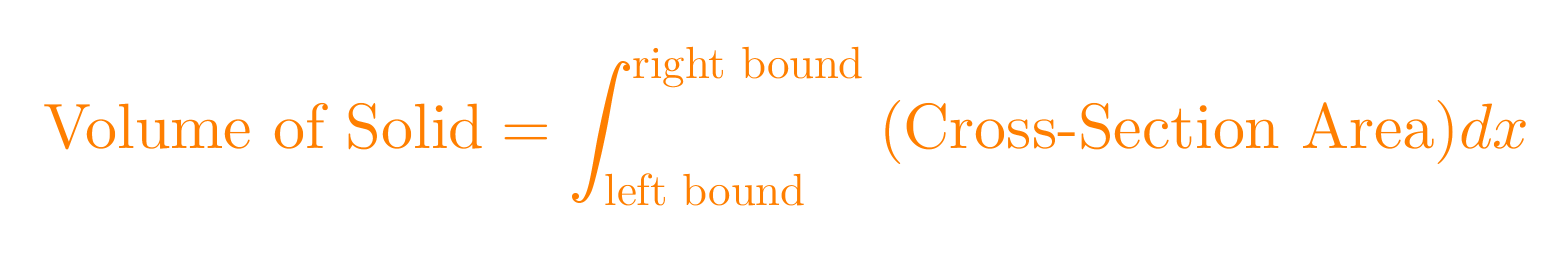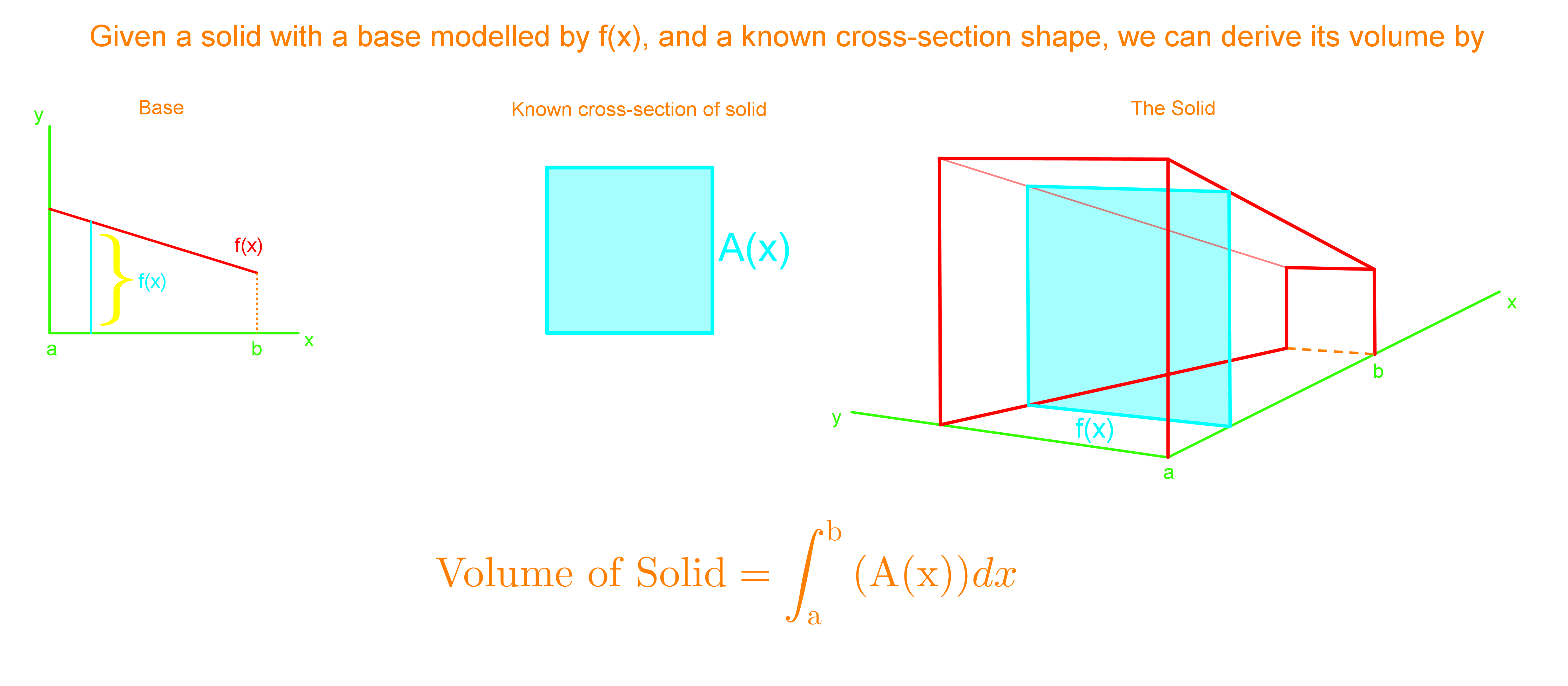# Volumes of solid with known cross-sections#### Everything You Need in One Place

Homework problems? Exam preparation? Trying to grasp a concept or just brushing up the basics? Our extensive help & practice library have got you covered.#### Learn and Practice With Ease

Our proven video lessons ease you through problems quickly, and you get tonnes of friendly practice on questions that trip students up on tests and finals.#### Instant and Unlimited Help

Our personalized learning platform enables you to instantly find the exact walkthrough to your specific type of question. Activate unlimited help now!

0/1
0/2
##### Examples
###### Lessons
1. A solid has a base bounded by $y=- {x\over2}+1$, $x=-2$,and $x=1$. If the parallel cross-sections perpendicular to the base are squares, find the volume of this solid.
1. A solid has a base bounded by these two curves, y= sin x, y= cos x. If the parallel cross-sections perpendicular to the base are equilateral triangles, find the volume of this solid.
###### Topic Notes
In this section, we learn that cross sections are shapes we get from cutting straight through the curve. We want to find the area of that cross section, and then integrate it with known bounds to find the volume of the solid. In most cases they will tell you what the shape of the cross-section is, so that you can find the area of cross-sections immediately.
Cross-Section Area of Solid = A(x)